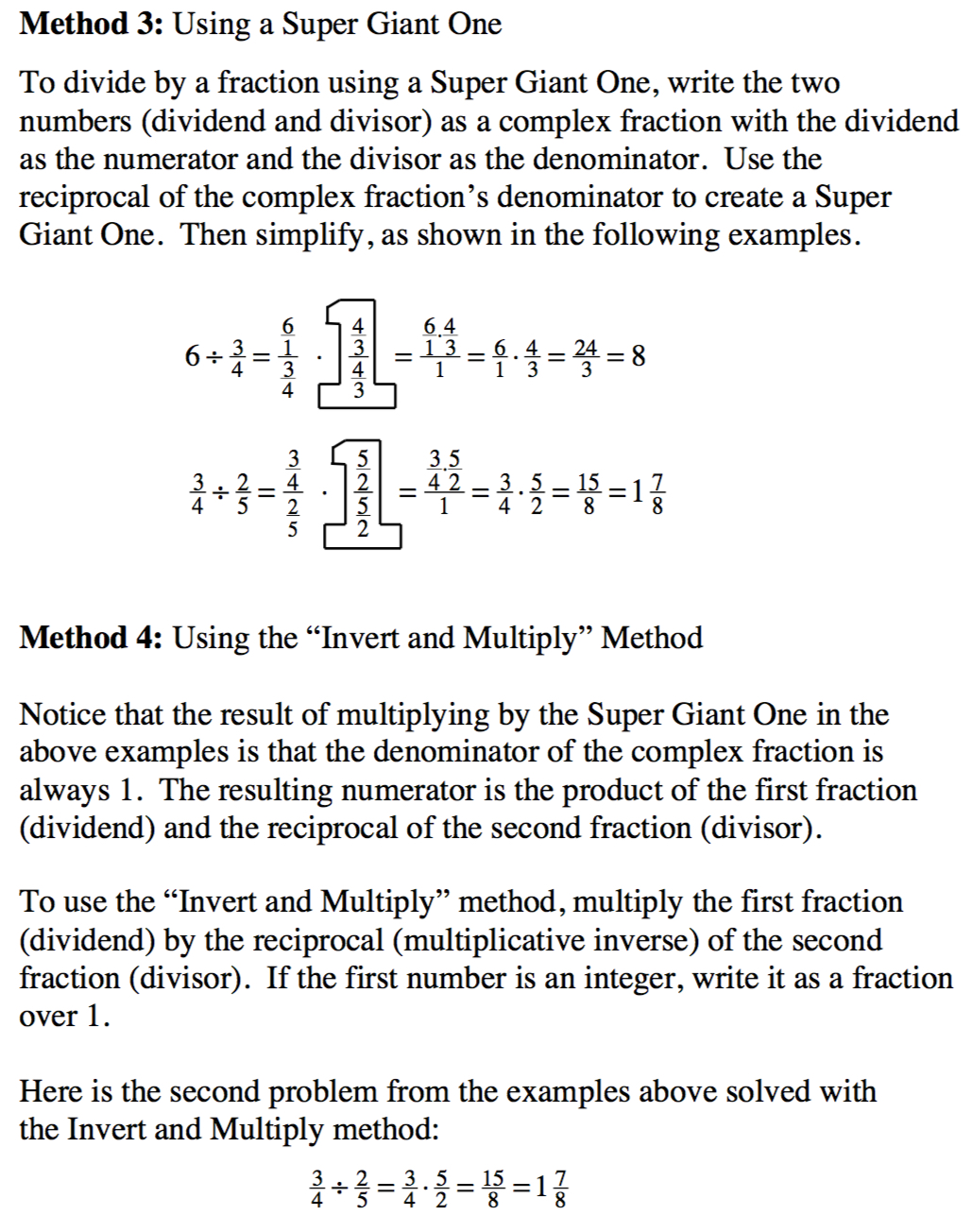### Home > CC1 > Chapter 7 > Lesson 7.2.4 > Problem7-74

7-74.

Simplify each of the following expressions by using one of the strategies in the Math Notes box for this lesson. Homework Help ✎

The Math Notes box is shown below for your reference.

1. $\frac { 2 } { 3 } \div \frac { 3 } { 5 }$

Make a complex fraction with the two fractions being divided.

$\frac{\frac{2}{3}}{\frac{3}{5}}$

Multiply by a "Super Giant One" to make the denominator equal to 1.
That is, multiply by a Giant One that uses the reciprocal of the denominator of the complex fraction.

$\frac{\frac{2}{3}}{\frac{3}{5}}\cdot \frac{\frac{5}{3}}{\frac{5}{3}}\\=\frac{\frac{2}{3}\cdot\frac{5}{3}}{1}\\=\frac{2}{3}\cdot \frac{5}{3}$

Finish by multiplying and simplifying.

$\frac{2}{3}\cdot\frac{5}{3}=\frac{10}{9}=1\frac{1}{9}$

1. $\frac { 5 } { 6 } \div \frac { 1 } { 12 }$

Follow the steps you used to solve part (a).

$10$

1. $3 \frac { 1 } { 8 } \div 2 \frac { 1 } { 2 }$

Write both mixed numbers as fractions greater than one, then write the expression as a complex fraction.